# RD Sharma Solutions for Class 6 Maths Chapter 3: Whole Numbers Objective Type Questions

Chapter 3 of RD Sharma Solutions is mainly about whole numbers where the natural numbers, including zero form the whole numbers, i.e. 0, 1, 2, 3, 4, 5, etc. The students can refer to RD Sharma Solutions Class 6, which boosts their confidence in solving problems in a shorter duration. The objective type questions are solved by experts with important formulas and concepts being highlighted, which makes it easier for the students during their exam preparation. RD Sharma Solutions for Class 6 Maths Chapter 3 Whole Numbers Objective Type Questions PDF are provided here for free download.

## RD Sharma Solutions for Class 6 Maths Chapter 3: Whole Numbers Objective Type Questions Download PDF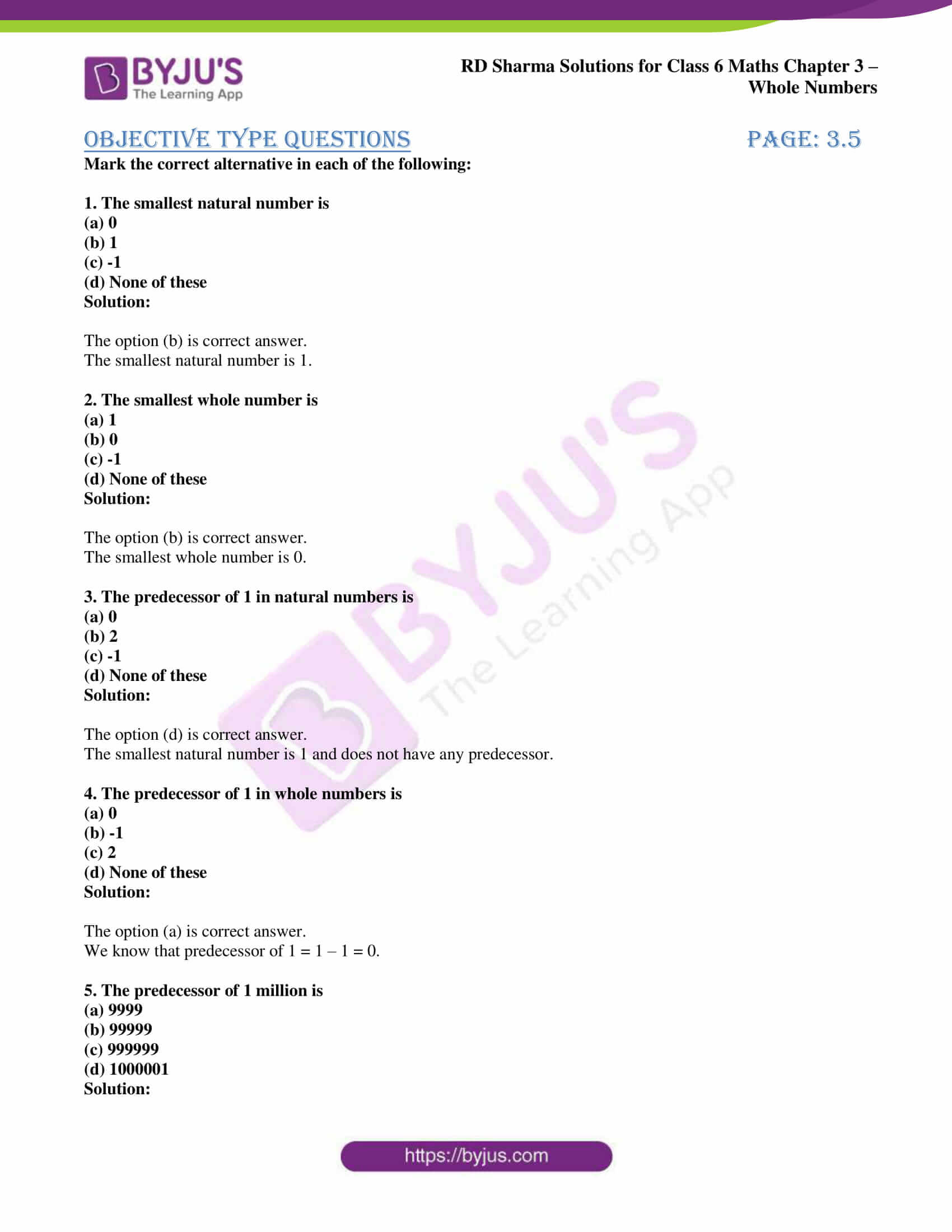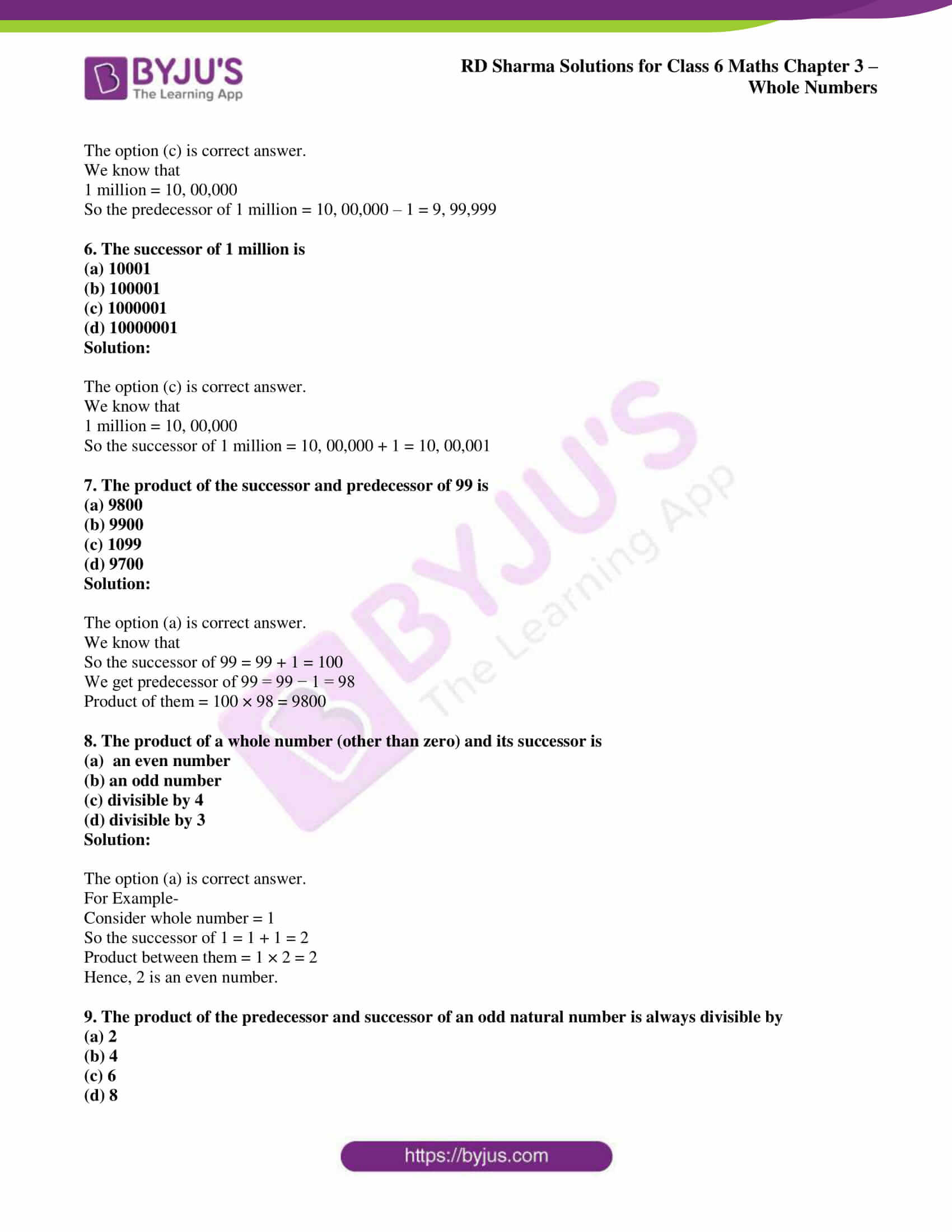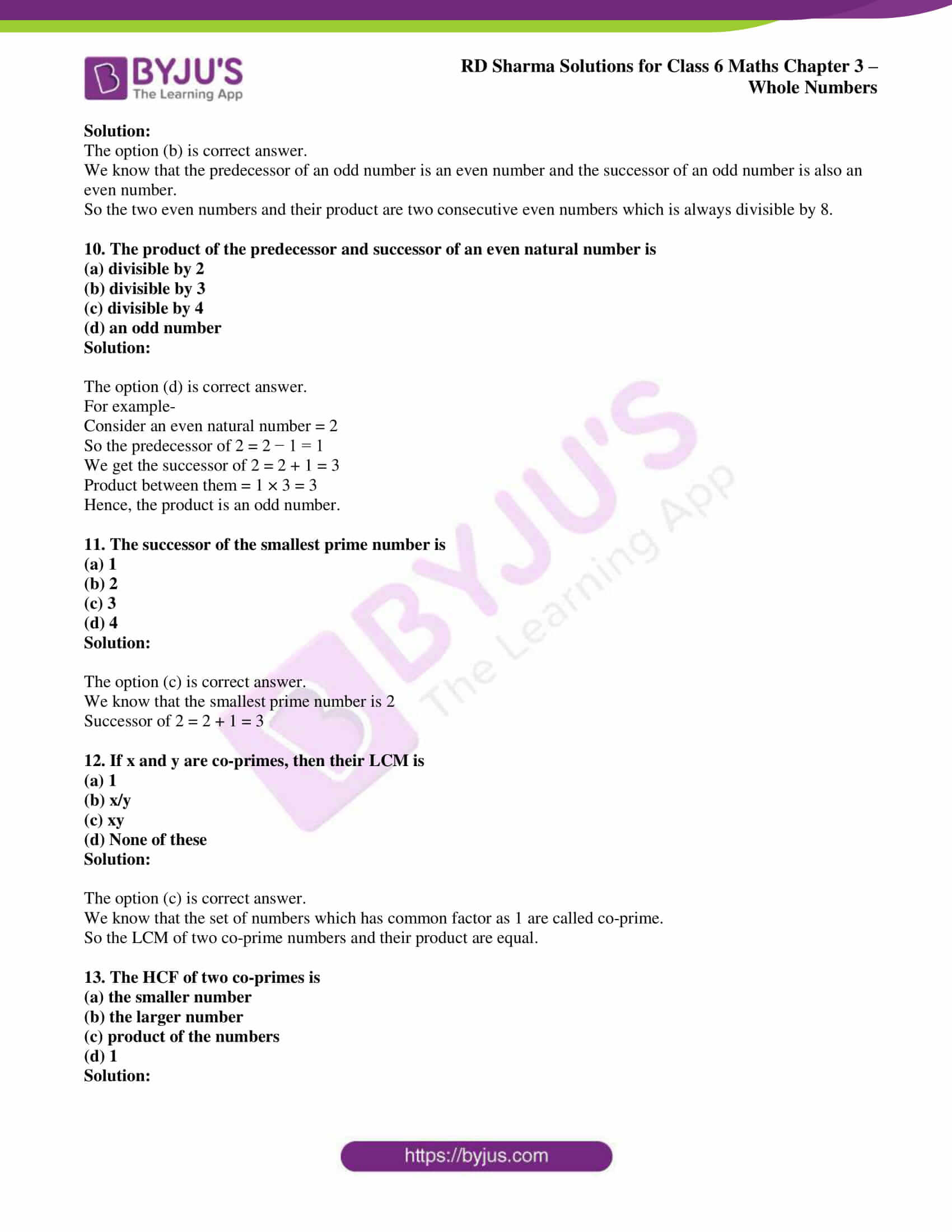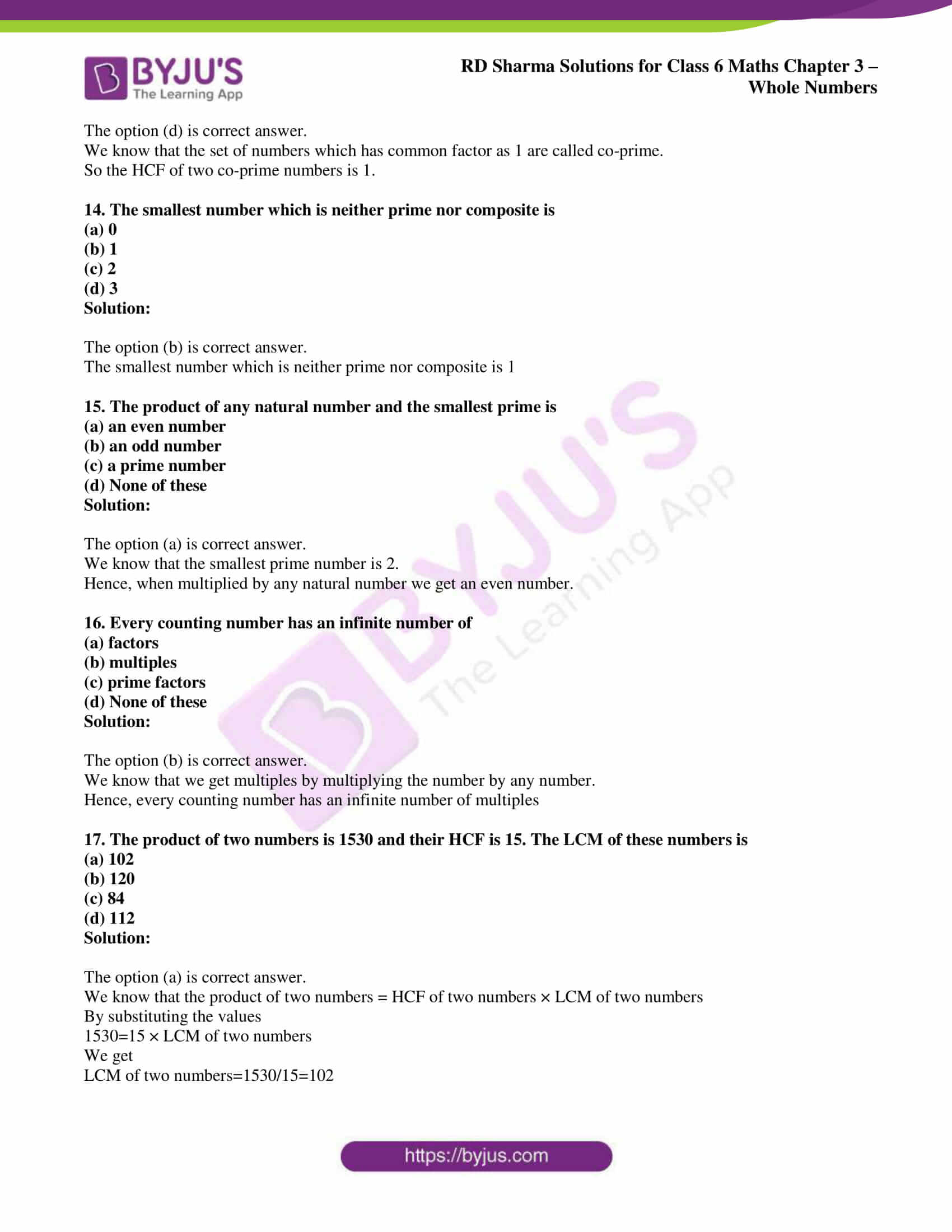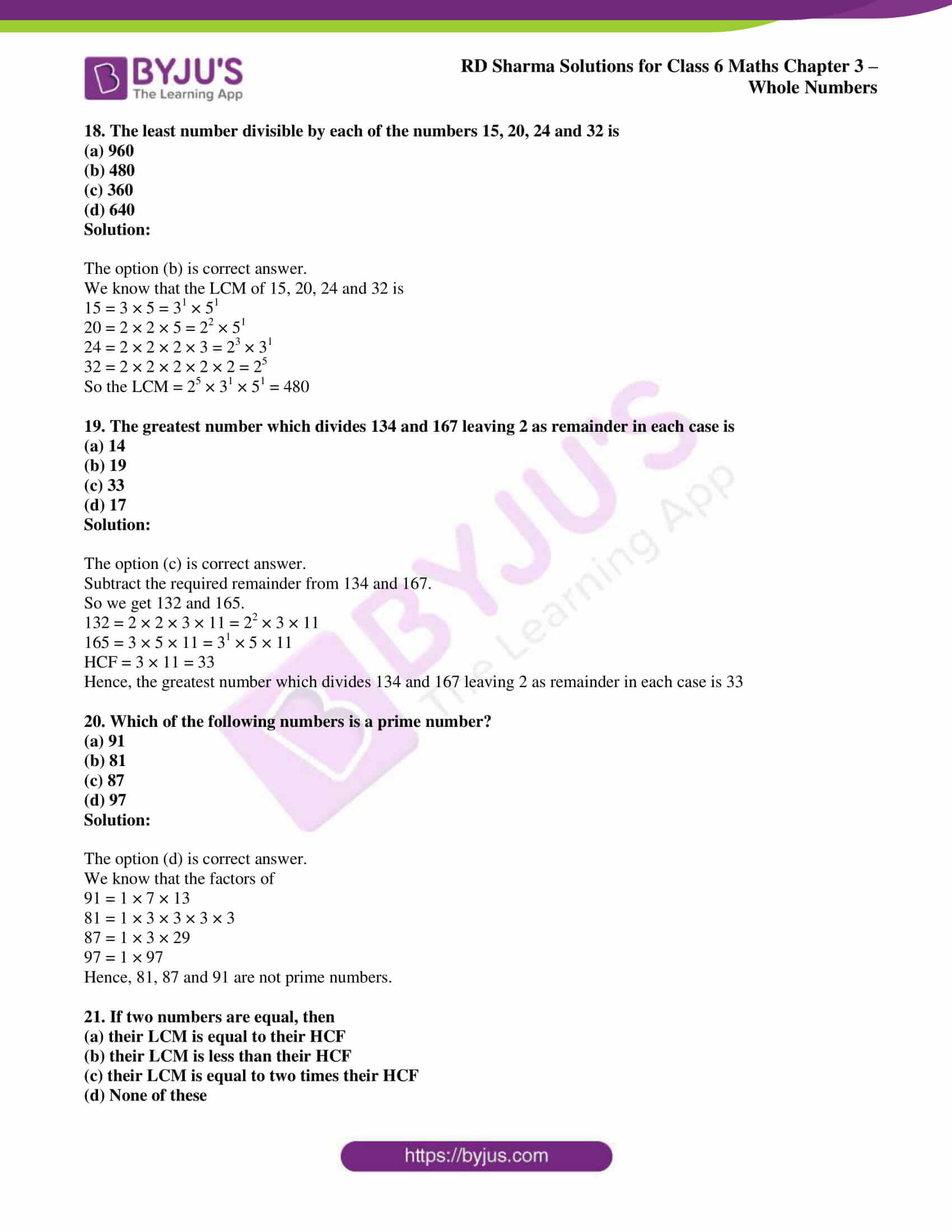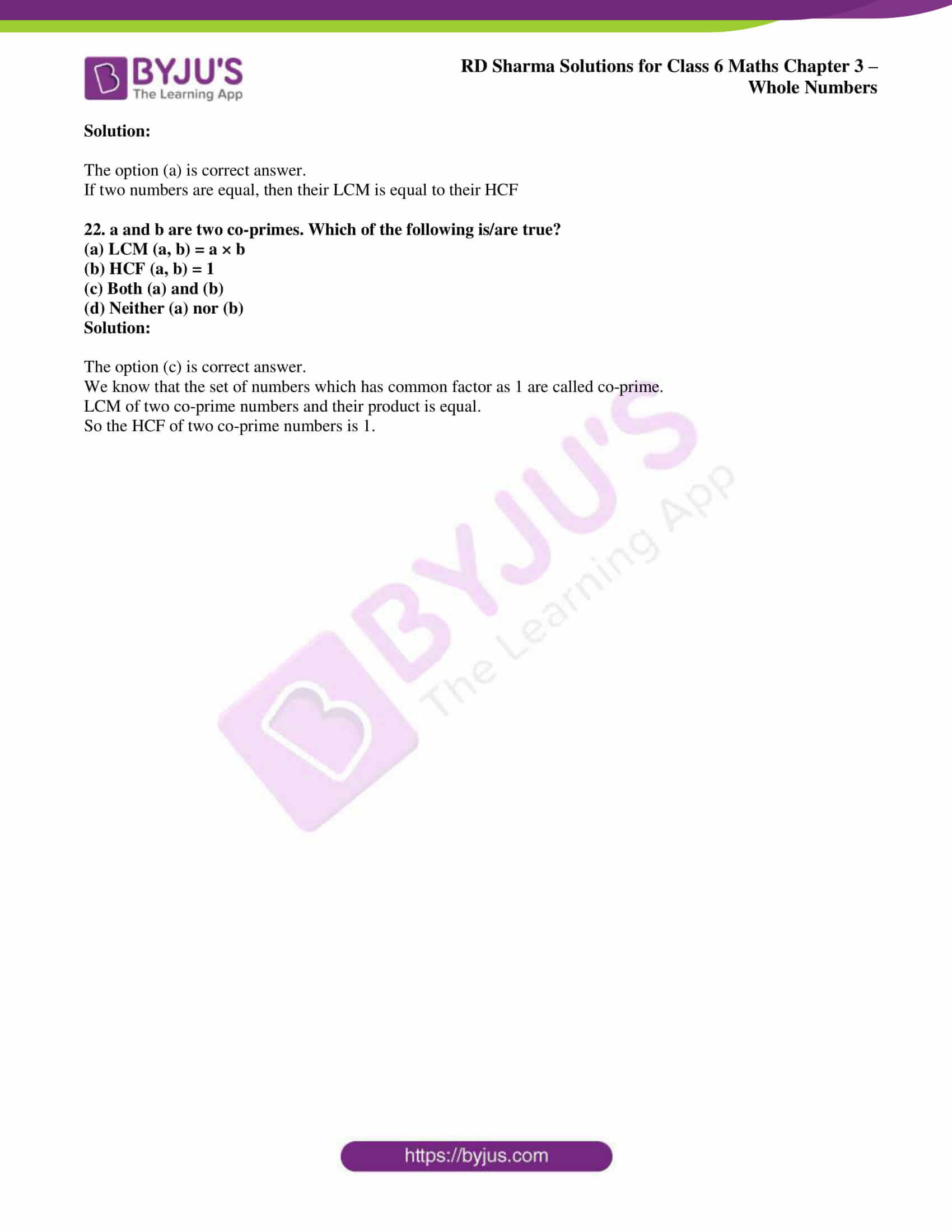### Access answers to Maths RD Sharma Solutions for Class 6 Chapter 3: Whole Numbers Objective Type Questions

Mark the correct alternative in each of the following:

1. The smallest natural number is
(a) 0
(b) 1
(c) -1
(d) None of these

Solution:

The option (b) is correct answer.

The smallest natural number is 1.

2. The smallest whole number is
(a) 1
(b) 0
(c) -1
(d) None of these

Solution:

The option (b) is correct answer.

The smallest whole number is 0.

3. The predecessor of 1 in natural numbers is
(a) 0
(b) 2
(c) -1
(d) None of these

Solution:

The option (d) is correct answer.

The smallest natural number is 1 and does not have any predecessor.

4. The predecessor of 1 in whole numbers is
(a) 0
(b) -1
(c) 2
(d) None of these

Solution:

The option (a) is correct answer.

We know that predecessor of 1 = 1 – 1 = 0.

5. The predecessor of 1 million is
(a) 9999
(b) 99999
(c) 999999
(d) 1000001

Solution:

The option (c) is correct answer.

We know that

1 million = 10, 00,000

So the predecessor of 1 million = 10, 00,000 – 1 = 9, 99,999

6. The successor of 1 million is
(a) 10001
(b) 100001
(c) 1000001
(d) 10000001

Solution:

The option (c) is correct answer.

We know that

1 million = 10, 00,000

So the successor of 1 million = 10, 00,000 + 1 = 10, 00,001

7. The product of the successor and predecessor of 99 is
(a) 9800
(b) 9900
(c) 1099
(d) 9700

Solution:

The option (a) is correct answer.

We know that

So the successor of 99 = 99 + 1 = 100

We get predecessor of 99 = 99 − 1 = 98

Product of them = 100 × 98 = 9800

8. The product of a whole number (other than zero) and its successor is
(a)  an even number
(b) an odd number
(c) divisible by 4
(d) divisible by 3

Solution:

The option (a) is correct answer.

For Example-

Consider whole number = 1

So the successor of 1 = 1 + 1 = 2

Product between them = 1 × 2 = 2

Hence, 2 is an even number.

9. The product of the predecessor and successor of an odd natural number is always divisible by
(a) 2
(b) 4
(c) 6
(d) 8

Solution:

The option (b) is correct answer.

We know that the predecessor of an odd number is an even number and the successor of an odd number is also an even number.

So the two even numbers and their product are two consecutive even numbers which is always divisible by 8.

10. The product of the predecessor and successor of an even natural number is
(a) divisible by 2
(b) divisible by 3
(c) divisible by 4
(d) an odd number

Solution:

The option (d) is correct answer.

For example-

Consider an even natural number = 2

So the predecessor of 2 = 2 − 1 = 1

We get the successor of 2 = 2 + 1 = 3

Product between them = 1 × 3 = 3

Hence, the product is an odd number.

11. The successor of the smallest prime number is
(a) 1
(b) 2
(c) 3
(d) 4

Solution:

The option (c) is correct answer.

We know that the smallest prime number is 2

Successor of 2 = 2 + 1 = 3

12. If x and y are co-primes, then their LCM is
(a) 1
(b) x/y
(c) xy
(d) None of these

Solution:

The option (c) is correct answer.

We know that the set of numbers which has common factor as 1 are called co-prime.

So the LCM of two co-prime numbers and their product are equal.

13. The HCF of two co-primes is
(a) the smaller number
(b) the larger number
(c) product of the numbers
(d) 1

Solution:

The option (d) is correct answer.

We know that the set of numbers which has common factor as 1 are called co-prime.

So the HCF of two co-prime numbers is 1.

14. The smallest number which is neither prime nor composite is
(a) 0
(b) 1
(c) 2
(d) 3

Solution:

The option (b) is correct answer.

The smallest number which is neither prime nor composite is 1

15. The product of any natural number and the smallest prime is
(a) an even number
(b) an odd number
(c) a prime number
(d) None of these

Solution:

The option (a) is correct answer.

We know that the smallest prime number is 2.

Hence, when multiplied by any natural number we get an even number.

16. Every counting number has an infinite number of
(a) factors
(b) multiples
(c) prime factors
(d) None of these

Solution:

The option (b) is correct answer.

We know that we get multiples by multiplying the number by any number.

Hence, every counting number has an infinite number of multiples

17. The product of two numbers is 1530 and their HCF is 15. The LCM of these numbers is
(a) 102
(b) 120
(c) 84
(d) 112

Solution:

The option (a) is correct answer.

We know that the product of two numbers = HCF of two numbers × LCM of two numbers

By substituting the values

1530=15 × LCM of two numbers

We get

LCM of two numbers=1530/15=102

18. The least number divisible by each of the numbers 15, 20, 24 and 32 is
(a) 960
(b) 480
(c) 360
(d) 640

Solution:

The option (b) is correct answer.

We know that the LCM of 15, 20, 24 and 32 is

15 = 3 × 5 = 31 × 51

20 = 2 × 2 × 5 = 22 × 51

24 = 2 × 2 × 2 × 3 = 23 × 31

32 = 2 × 2 × 2 × 2 × 2 = 25

So the LCM = 25 × 31 × 51 = 480

19. The greatest number which divides 134 and 167 leaving 2 as remainder in each case is
(a) 14
(b) 19
(c) 33
(d) 17

Solution:

The option (c) is correct answer.

Subtract the required remainder from 134 and 167.

So we get 132 and 165.

132 = 2 × 2 × 3 × 11 = 22 × 3 × 11

165 = 3 × 5 × 11 = 31 × 5 × 11

HCF = 3 × 11 = 33

Hence, the greatest number which divides 134 and 167 leaving 2 as remainder in each case is 33

20. Which of the following numbers is a prime number?
(a) 91
(b) 81
(c) 87
(d) 97

Solution:

The option (d) is correct answer.

We know that the factors of

91 = 1 × 7 × 13

81 = 1 × 3 × 3 × 3 × 3

87 = 1 × 3 × 29

97 = 1 × 97

Hence, 81, 87 and 91 are not prime numbers.

21. If two numbers are equal, then
(a) their LCM is equal to their HCF
(b) their LCM is less than their HCF
(c) their LCM is equal to two times their HCF
(d) None of these

Solution:

The option (a) is correct answer.

If two numbers are equal, then their LCM is equal to their HCF

22. a and b are two co-primes. Which of the following is/are true?
(a) LCM (a, b) = a × b
(b) HCF (a, b) = 1
(c) Both (a) and (b)
(d) Neither (a) nor (b)

Solution:

The option (c) is correct answer.

We know that the set of numbers which has common factor as 1 are called co-prime.

LCM of two co-prime numbers and their product is equal.

So the HCF of two co-prime numbers is 1.